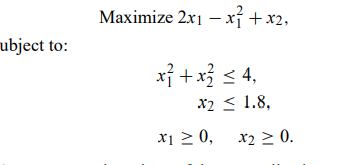# Consider the nonlinear-programming problem

Consider the nonlinear-programming problem:a) Carry out two iterations of the generalized programming algorithm on this problem. Le

t C = {(x1, x2)|x1 ≥ 0, 0 ≤ x2 ≤ 1.8},

leaving only one constraint to handle explicitly. Start with the following two initial candidate solutions:

x1 = 0, x2 = 1.8, and x1 = 2, x2 = 0.

Is the solution optimal after these two iterations?

b) Carry out two iterations of the MAP algorithm on the same problem. Start with x1 = 0, x2 = 0, as the initial solution, and set the parameters δ1 = δ2 = 2 at iteration 1 and δ1 = δ2 = 1 at iteration 2. Is the solution optimal after these two iterations?